Courses

# Vector Algebra Physics Notes | EduRev

## Physics : Vector Algebra Physics Notes | EduRev

The document Vector Algebra Physics Notes | EduRev is a part of the Physics Course Basic Physics for IIT JAM.
All you need of Physics at this link: Physics

Introduction
Vector is one of the most important for studying physics. But to know about vectors you should first know about a scalar. A scalar is a quantity (length etc) which has only the magnitude (3 m or 4 m and so on) but it doesn't specify the direction as in 3 m to the south of a reference point on a reference plane. This is where ventor comes in use as most of the quantity defined in physics involves direction along with the magnitude.
Vector follows the rules of mathematics and geometry as well just in a different manner as the direction pokes its nose the basic concept. And while adding the magnitude of the vectors we are also supposed to keep in mind the direction. In the following paragraph, you will learn to 'visualise' the concept as its he key aim of physics – to be able to 'see' things and not just mathematically or practically prove it.

Before we dig deeper into the mathematical concepts, we first need to clarify that, vector deals only with the starting and the end points and not the path covered. There in the figure 1, there are several ways to go to point C from A via B, but the vector is meant to deal with the shortest path, that is the straight lines joining them.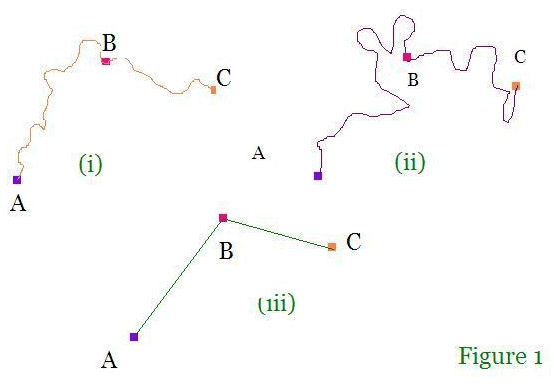If we want to add two vectors, there are two pairs of starting and ending points, and in order to add them, we need to move the second vector's starting point to the end point of the frist vector without changing either of them's direction, and thus as a resultant we get two point in total which is the starting point of the first vector (which becomes starting point of the resultant vector) and the end point of the second vector (which becomes the end point of the resultant vector). And then the shortest path from the starting to the end point of the resultant vector gives the complete resultant vector. The concept is illustrated in the figure 2.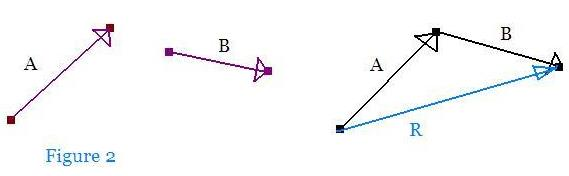Similarly we can add three vectors and more subsequently as in Figure 3.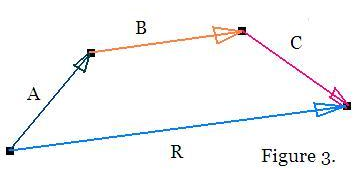Vector subtraction
Vector subtraction becomes a little tricky but uses the same concept as the addition, except that the direction of the subtrant is 'reversed' as in the starting and the end points of the second vectors interchange their positions and point in the opposite direction, and simultaneously, a minus (–) sign is added in front of the second vector. The concept is illustrated in Figure 4.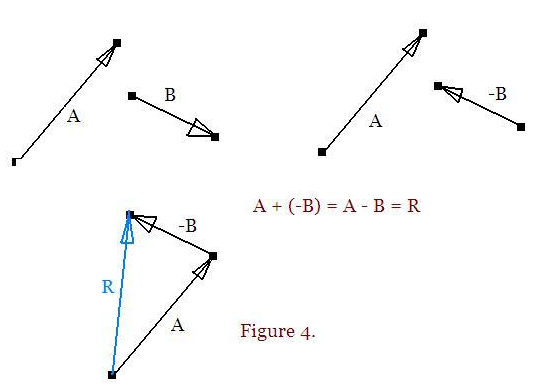Vector multiplication
Vector multiplication is very different from scalar multiplication and it involves a further concept of resolving the vector into its components which is explained in the following section.

Resolving a vector into components
Resolving a vector into components is more understood visually than mathematically. Here we take an example of  a vector that is lying at angle (theta)with respect to the horizontal reference. And we can picture the horizontal component of the vector if we place a light source in the vertical position looking down at the vector and the shadow that falls on the horizontal line is the required horizontal component of the vector. It's a scalar and it doesn't have a direction. Similarly the vertical component can be evaluated if we place the same light source on the left of the vector with its shadow falling on the vertical line. It is again a scalar quantity. The whole concept is illustrated in the Figure 5.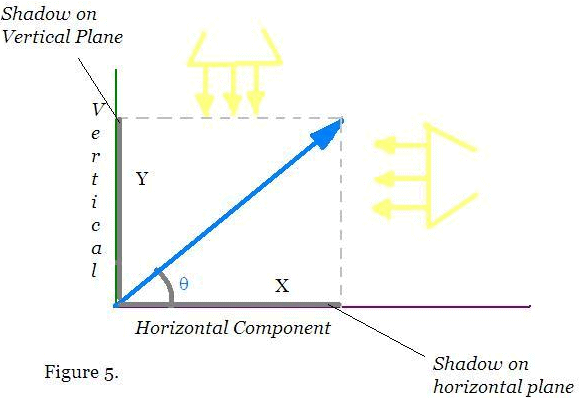Now based on the previously discussed concept we can see that cos (theta) = X/A and sin (theta) = Y/A
Therefore, the horizontal component X = Acos(theta) and horizontal component Y = Asin(theta)

Scalar (or 'dot') products
The scalar product (also called 'dot' product) is operated on two vectors at a time. If we require to find out the dol product of the two vector, we move the starting point of any one of the vectors and we align the starting points of the vectors so that they have the common starting point and without changing the direction of either. Now we find that there is some angle between the two vectors (it can also be 0) and the process of multiplication is done by multiplying one of the vectors with the horizontal component of the other (as we learnt in the previous section, i.e. we multiply one of the vectors with the shadow of the other vector over it). We can choose either one to be static and take the component of the other. This is illustrated in the Figure 6.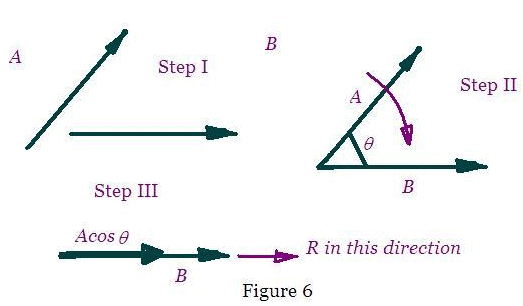Note: Scalar product is commutative

Vector (or 'cross') products
Finding vector or cross products follow the initial steps of finding the dot products. We align the starting points of the vectors and then, we rotate one of the vectors (direction of the vectors are tampered with) to make it align with the other vector and the resultant vector follows the 'screw driver rule'. That is, if you rotate the vector counter clockwise for the purpose of aligning it with the other, then the resultant vector' comes out of the plane, the direction of the vector is perpendicular to the plane of the paper and towards your face (just like the screw coming out of the board) and similarly when you rotate it in the clockwise direction, the vector goes 'inside' the plane and the direction of the vector is away from ur face. This is illustrated in the Figure 7.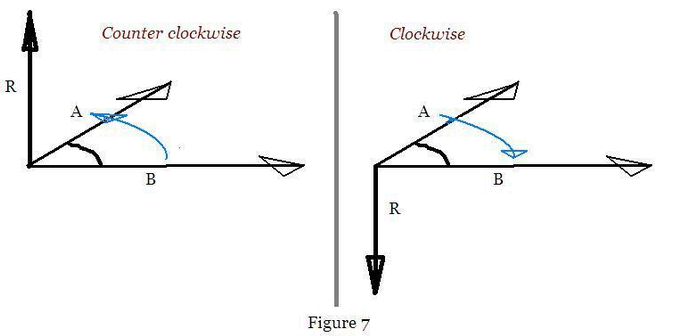The formula for the vector product for two vectors A and B is A X B = ABsin(theta)

Note: it is obvious that vector product is not commutative or associative as A X B is not equal to BXA. Even if the magnitudes are the same, the directions are straight angle opposite.

Vectors are the most handy tools to work with in advanced geometry and physics and sometimes chemistry and several other fields of study. It is one of the basic building blocks of science and thus should be clearly understood before proceeding.

Offer running on EduRev: Apply code STAYHOME200 to get INR 200 off on our premium plan EduRev Infinity!

,

,

,

,

,

,

,

,

,

,

,

,

,

,

,

,

,

,

,

,

,

;